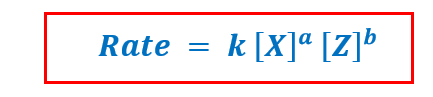# Problem: The following kinetic data are collected for the initial rates of a reaction 2X + Z →products:Experiment  [X]0  (M)  [Z]0  (M)  Rate  (M/s) 10.250.254.0x101imes {10^{1}}20.500.503.2x102imes {10^{2}}30.500.757.2x102imes {10^{2}}What is the value of the rate constant with proper units?

###### FREE Expert Solution

Recall that the Rate Law (which considers reactants only) for the reaction:

2X + Z → products is given by the equation:Where:

k = rate law constant

a, b = reactant orders

X and Z = reactants

Based on the given experimental data, we can determine the rate constant, k by these steps:

1. Solve reactant orders: a and b
2. Solve for the value of k
3. Determine correct units for k
83% (276 ratings)###### Problem Details

The following kinetic data are collected for the initial rates of a reaction 2X + Z →products:

 Experiment [X]0  (M) [Z]0  (M) Rate  (M/s) 1 0.25 0.25 4.0x101 2 0.50 0.50 3.2x102 3 0.50 0.75 7.2x102

What is the value of the rate constant with proper units?

Frequently Asked Questions

What scientific concept do you need to know in order to solve this problem?

Our tutors have indicated that to solve this problem you will need to apply the Rate Law concept. You can view video lessons to learn Rate Law. Or if you need more Rate Law practice, you can also practice Rate Law practice problems.

What professor is this problem relevant for?

Based on our data, we think this problem is relevant for Professor Sudhakaran's class at Del Mar College.